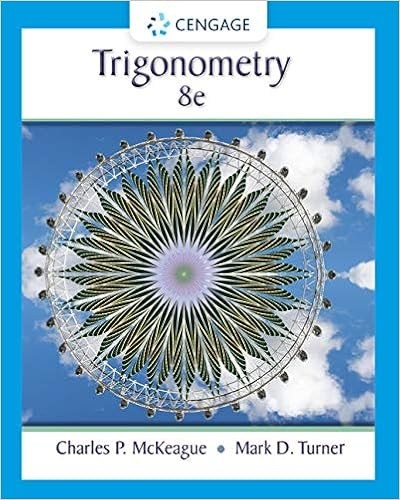• Notes
• 210
• 84% (19) 16 out of 19 people found this document helpful

This preview shows page 80 - 82 out of 210 pages.

##### We have textbook solutions for you!
The document you are viewing contains questions related to this textbook.The document you are viewing contains questions related to this textbook.
Chapter 3 / Exercise 22
Trigonometry
McKeague/TurnerExpert Verified
Explain your answer. (c) Take your routine for doing Newton’s method and modify it to run the secant method. Repeat the run of part (b), using, say, x 0 = 4 and x 1 = 5, and again predict (without running the code further) how many steps would be required to reduce | f ( x k ) | below 10 16 (assuming that your machine carried enough decimal places to do this) using the secant method. Explain your answer. 3. Newton’s method can be used to compute reciprocals, without division. To compute 1 /R , let f ( x ) = x 1 R so that f ( x ) = 0 when x = 1 /R . Write down the Newton iteration for this problem and compute (by hand or with a calculator) the first few Newton iterates for approximating 1 / 3, starting with x 0 = 0 . 5, and not using any division. What happens if you start with x 0 = 1? For positive R , use the theory of fixed point iteration to determine an interval about 1 /R from which Newton’s method will converge to 1 /R . 80
##### We have textbook solutions for you!
The document you are viewing contains questions related to this textbook.The document you are viewing contains questions related to this textbook.
Chapter 3 / Exercise 22
Trigonometry
McKeague/TurnerExpert Verified
4. Use Newton’s method to approximate 2 to 6 decimal places. 5. Write down the first few iterates of the secant method for solving x 2 3 = 0, starting with x 0 = 0 and x 1 = 1. 6. Consider the function h ( x ) = x 4 / 4 3 x . In this problem, we will see how to use Newton’s method to find the minimum of the function h ( x ). (a) Derive a function f that has a root at the point where h achieves its minimum. Write down the formula for Newton’s method applied to f . (b) Take one step (by hand) with Newton’s method starting with a guess of x 0 = 1. (c) Take two steps (by hand) towards the root of f (i.e., the minimum of h ) using bisection with a = 0, b = 4. 7. In finding a root with Newton’s method, an initial guess of x 0 = 4 with f ( x 0 ) = 1 leads to x 1 = 3. What is the derivative of f at x 0 ? 8. In using the secant method to find a root, x 0 = 2, x 1 = 1 and x 2 = 2 with f ( x 1 ) = 4 and f ( x 2 ) = 3. What is f ( x 0 )? 9. Can the bisection method be used to find the roots of the function f ( x ) = sin( x ) + 1? Why or why not? Can Newton’s method be used to find the roots (or a root) of this function? If so, what will be its order of convergence and why? 10. The function f ( x ) = x 2 2 x + 1 x 2 x 2 has exactly one zero in the interval [0 , 3], at x = 1. Using a = 0 and b = 3, run the bisection method on f ( x ) with a stopping tolerance of delta = 1e-3 . Explain why it does not appear to converge to the root. Why does the Intermediate Value Theorem (3.1.1) not guarantee that there is a root in [0 , 1 .
•••